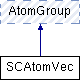ParM  parm A molecular dynamics library
SCAtomVec Class Reference

#include <interaction.hpp>

Inheritance diagram for SCAtomVec:Public Member Functions

SCAtomVec (vector< double > masses)

SCAtomVec (uint N, flt mass)

AtomVecvec ()

Atomoperator[] (cuint n)

Atomoperator[] (cuint n) const

AtomID get_id (cuint n)

IDPair pair (cuint n)

uint size () const
Number of atoms in the group. More...

uint pairs () const

~SCAtomVec ()Public Member Functions inherited from AtomGroup
virtual Atomget (cuint n)

virtual AtomIter begin ()
For use in a for loop. More...

virtual AtomIter end ()

Vec com () const
center of mass More...

Vec com_force () const
center of mass force (i.e., sum of all forces) More...

Vec com_velocity () const
center of mass velocity More...

flt mass () const
Mass of the whole group. More...

flt kinetic_energy (const Vec originvelocity=Vec::Zero()) const
Total kinetic_energy energy of the group. More...

Vec momentum () const
Total momentum. More...

$$R_g$$ More...

Vec torque (const Vec loc) const
Total torque about a given location. More...

Vec torque () const

flt moment_about (const Vec axis, const Vec loc) const
Moment of inertia of the atoms about an axis. More...

flt moment_about (const Vec axis) const

Vec angular_momentum (const Vec loc) const
Angular momentum. More...

Vec angular_momentum () const

Matrix moment (const Vec loc) const
Moment of inertia of the atoms as a whole. More...

Matrix moment () const

Vec omega (const Vec loc) const
Angular velocity. More...

Vec omega () const

void add_omega (Vec w, Vec origin)
Add a given angular velocity to all atoms, by adding to their velocity. More...

void reset_L ()
Reset angular momentum to 0. More...

for resetting. Adds a fixed velocity to all atoms More...

void reset_com_velocity ()
Subtracts the center of mass velocity from all atoms. More...

void randomize_velocities (flt T)
Randomize velocities, for a specific temperature. More...

void reset_forces ()
for timestepping More...

virtual ~AtomGroup ()

Static Public Member Functions

static flt volume (flt diameter, flt length, uint dim=NDIM)
The volume of a single spherocylinder diameter: the width of the spherocylinder length: the end-to-end length. More...

Constructor & Destructor Documentation

 SCAtomVec::SCAtomVec ( vector< double > masses )
inline
 SCAtomVec::SCAtomVec ( uint N, flt mass )
inline
 SCAtomVec::~SCAtomVec ( )
inline

Member Function Documentation

 AtomID SCAtomVec::get_id ( cuint n )
inlinevirtual

Implements AtomGroup.

 Atom& SCAtomVec::operator[] ( cuint n )
inlinevirtual

Implements AtomGroup.

 Atom& SCAtomVec::operator[] ( cuint n ) const
inlinevirtual

Implements AtomGroup.

 IDPair SCAtomVec::pair ( cuint n )
inline
 uint SCAtomVec::pairs ( ) const
inline
 uint SCAtomVec::size ( ) const
inlinevirtual

Number of atoms in the group.

Implements AtomGroup.

 AtomVec& SCAtomVec::vec ( )
inlinevirtual

Implements AtomGroup.

 flt SCAtomVec::volume ( flt diameter, flt length, uint dim = NDIM )
static

The volume of a single spherocylinder diameter: the width of the spherocylinder length: the end-to-end length.

The documentation for this class was generated from the following files: